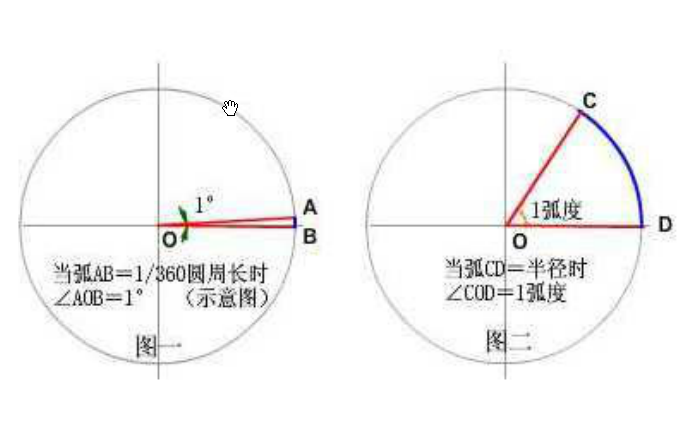# 笔记：Flash & AS3 中的弧度和角度

## 1.角的两种单位

「弧度「和「度」是度量角的大小的两种不同单位。就像「米」和「市尺」是度量长度大小的两种不同单位一样。

## 2.弧度的定义:

### 「弧度」的定义:``角（弧度） = 弧长/半径``
• 园的周长是半径的2π倍，所以一个周角（360度）就是 2π 弧度;
• 半圆的长度是半径的π倍，所以一个平角（180度）是 π 弧度。

## 3.度与弧度之间的转换：

``````function angleToRadian(angle:Number):Number{
return angle*(Math.PI/180);
}

}

function sinD(angle:Number):Number{
}

function cosD(angle:Number):Number{
}

function atan2D(y:Number,x:Number):Number{